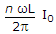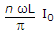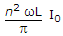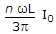# Electronics and Communication Engineering - Power Electronics

### Exercise :: Power Electronics - Section 4

6.

Which converter is used in HVDC transmission?

 A. 3 pulse B. 6 pulse C. 12 pulse D. 24 pulse

Explanation:

No answer description available for this question. Let us discuss.

7.

Assertion (A): The turn on time of SCR is about 1 to 4 ms.

Reason (R): The turn off time of SCR is about 10 to 300 ms.

 A. Both A and R are correct and R is correct explanation of A B. Both A and R correct but R is not correct explanation of A C. A is correct but R is wrong D. A is wrong but R is correct

Explanation:

No answer description available for this question. Let us discuss.

8.

An n pulse rectifier is fed by a source having an inductance L. If load current is I0, the voltage drop due to overlap is

 A.B.C.D.Explanation:

No answer description available for this question. Let us discuss.

9.

Which chopper circuit operates in all the 4 quadrants?

 A. A B. C C. D D. E

Explanation:

No answer description available for this question. Let us discuss.

10.

A single phase cycloconverter feeds a resistance heating load. The heating power is provided by

 A. only the fundamental component of output wave B. only higher harmonics in the output wave C. both fundamental and higher harmonics in the output wave D. either (a) or (b)